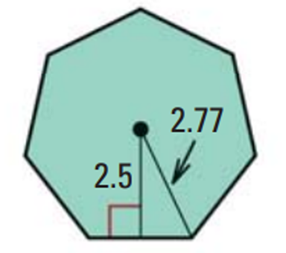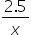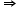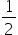Mathematics
Easy

Question

# Find the area of the regular polygon.## 35    32    21    24Hint:

## The correct answer is: 21

### In the question there is a polygon as shown in the figure above.Here, we have to find the area of the given polygon.From the figure we can say that, apothem= 2.5Firstly, we have to find the length of each side of the polygon.For that, let us consider length of the polygon be x.So, tan 64.29 =x = 1.2Then, length of each side(a) = 2×1.2 = 2.4Perimeter(P) = 2.4 × 7 = 16.8A =×a ×P=×2.5 × 16.8= 21So, the area of the given polygon is 21.Therefore, the correct option is c, i.e., 21.

A perpendicular line segment joining the centre and one of the sides of the regular polygon is called apothem of the polygon.

### Related Questions to study#### With Turito Foundation.#### Get an Expert Advice From Turito.